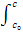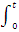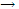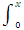Explain Second Order Rate Equations.

Integration of the second order rate equations also produces convenient expressions for dealing with concentration time results.

A reaction is classified as second order if the rate of the reaction is proportional to the square of the concentration of one of the reagents or to the product of the concentrations of two species of the reagents. The second situation leads to the same equations as the first if the two reactants are used up at the same rate and if their initial concentrations are equal.

Rate = kc2. For these situations, the rate equation is

-dc/dt = kc2, where c is the concentration of the single reagent or one of the two reagents. Again the kinetic data are usually compared with the integrated form of the equation. One has

-dc/c2 = kdt

And

1/c - 1/c0 = kt

A reaction of the types considered so far is therefore second order if a plot of 1/c versus t gives a straight line. The slope of the straight line is equal to the rate constant. Equation shows, this constant involves the units of concentrations, differing in this respect from the first order rate constant that involves only the units of time. Furthermore, the time for the concentration to drop to half its initial value is deduced from equation to be

t1/2 = 1/kc
0

The half life therefore depends on the initial concentration and is not a convenient way of expressing the rate constant of second order reactions.

Example: use of second order half life expression to verify that the rates used which were obtained for periods of reaction up to about 30 min, were indeed "initial rates".

Solution:
although the concentrations of the two reactants, iodine ions and peroxydisulfate ions, reported are somewhat different we can use a representative value of 0.01 mol L-1. Then with k = 0.36 mol-1 L min-1, gives

t1/2 = 1/(0.36 mol-1 L min-1) (0.01 mol L-1)

= 300 min

About half the reactants would be used up in 300 min. since the reaction was studied only in times up to about 10 min, the initial concentration could not have changed appreciably. The method of initial rates was applicable.

Rate = kcA cB: instead of working with the concentration of the reacting species, as was done with previous equation, it is more convenient to develop the rate equation by introducing a term for the amount of reaction that has occurred at time t. the overall reaction might, for example, be of the form

A + Bproducts

If it is inconvenient to arrange to have the initial concentrations of A and B equal the analysis that led to equation cannot be used, but the kinetic data can be treated in terms of the following quantities:

a = initial concentration of A

b = initial concentration of B

X = decrease in A or B at time t = amount of product at time t

a - x = concentration of A at time t

b - x = concentration of B at time t

The differential second order rate equation would then be

dx/dt = k[A][B] = k(a - x)(b - x)

The integration can be performed by using partial fractions. Thus

dx/(a - x)(b - x) = k dt

1/a - b(- dx/a - x + dx/b - x) = kdt

On integration this gives

1/a - b [In (a - x) - In (b - x)]0x = kt

Insertion of the limits and rearrangement give, finally,

1/a - b In b(a - x)/a(b - x) = kt

#### Related Questions in Chemistry

• ##### Q :Problem based on molecular weight

Select the right answer of the question. Molecular weight of urea is 60. A solution of urea containing 6g urea in one litre is : (a)1 molar (b)1.5 molar (c) 0.1 molar (d) 0.01 molar

• ##### Q :What is electrolytic dissociation? The

The Debye Huckel theory shows how the potential energy of an ion in solution depends on the ionic strength of the solution.Except at infinite dilution, electrostatic interaction between ions alters the properties of the solution from those excepted from th

• ##### Q :Question related to colligative

The colligative properties of a solution depend on: (a) Nature of solute particles present in it (b) Nature of solvent used (c) Number of solute particles present in it (d) Number of moles of solvent only

• ##### Q :Raoults law Give me answer of this

Give me answer of this question. Provide solution of this question. Which one of the following is the expression of Raoult's law: (a) P-P1/P = n/n+N (b) P1-P/P = N/ N+n (c)P-P2/P1= N/ N-n (d) P1-P/P2= N-n/N

• ##### Q :Basicity order order of decreasing

order of decreasing basicity of urea and its substituents

• ##### Q :Composition of the vapour Choose the

Choose the right answer from following. An ideal solution was obtained by mixing methanol and ethanol. If the partial vapour pressure of methanol and ethanol are 2.619KPa and 4.556KPa respectively, the composition of the vapour (in terms of mole fraction) will be: (

• ##### Q :Explain various chemicals associated

During processing of food, several chemicals are added to it to augment its shelf life and to make it more attractive as well. Main types of food addi

• ##### Q :Question associated to vapour pressure

Choose the right answer from following. The vapour pressure lowering caused by the addition of 100 g of sucrose(molecular mass = 342) to 1000 g of water if the vapour pressure of pure water at 25degree C is 23.8 mm Hg: (a)1.25 mm Hg (b) 0.125 mm Hg (c) 1.15 mm H

• ##### Q :Solubility are halides are halogens

are halides are halogens more soluble? why?

• ##### Q :Problem on convection coefficient An

An experiment to determine the convection coefficient associated with airflow over the surface of a thick stainless steel casting involves insertion of thermocouples in the casting at distances of 10 mm and 20 mm from the surface.  When the experiment was perform